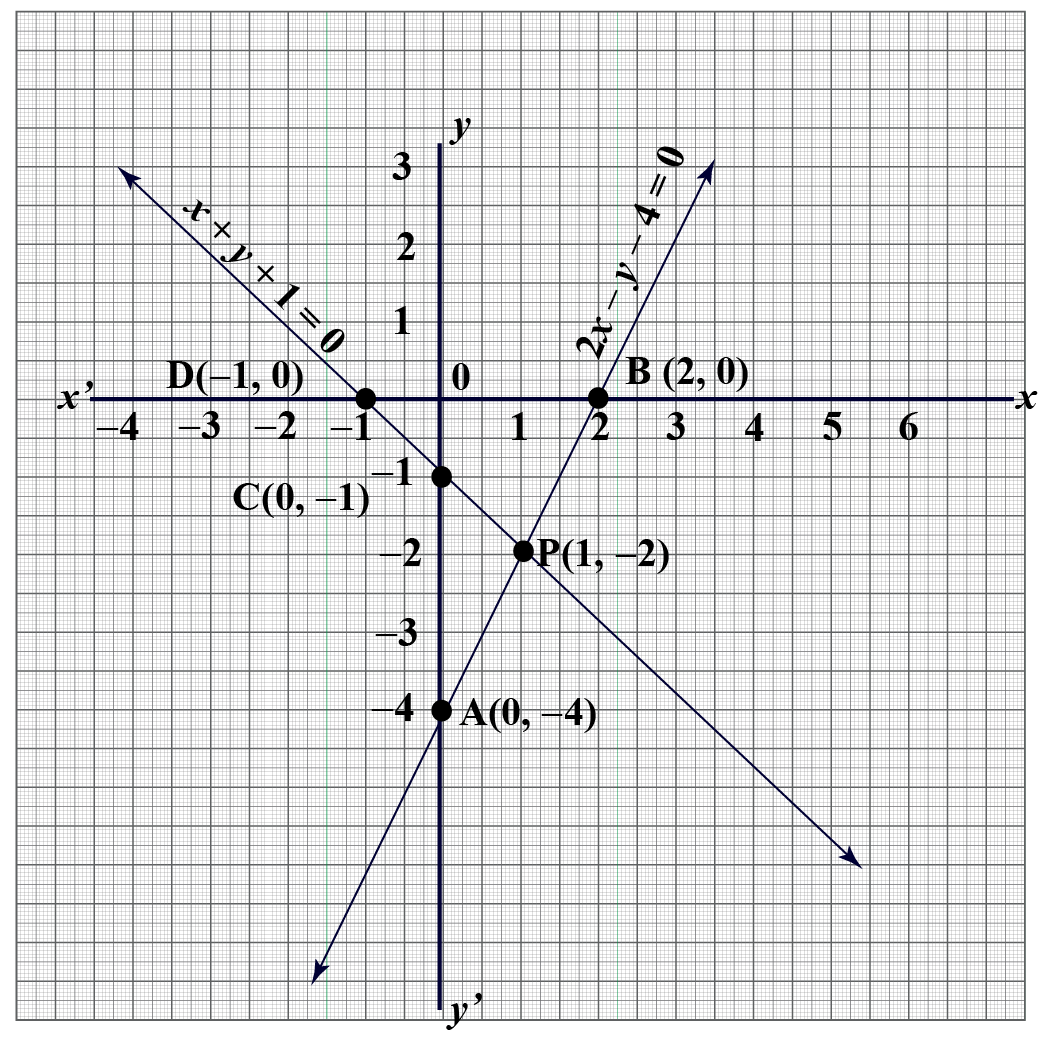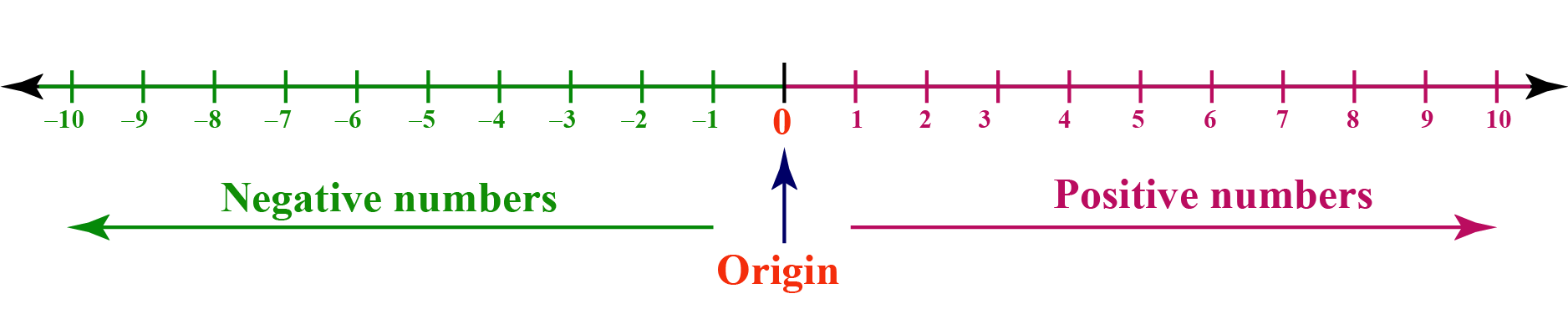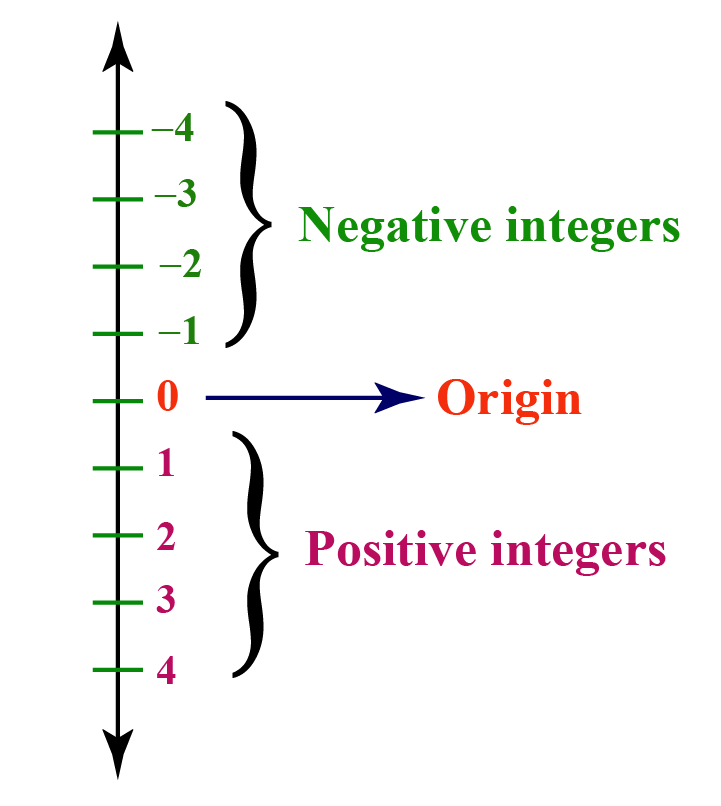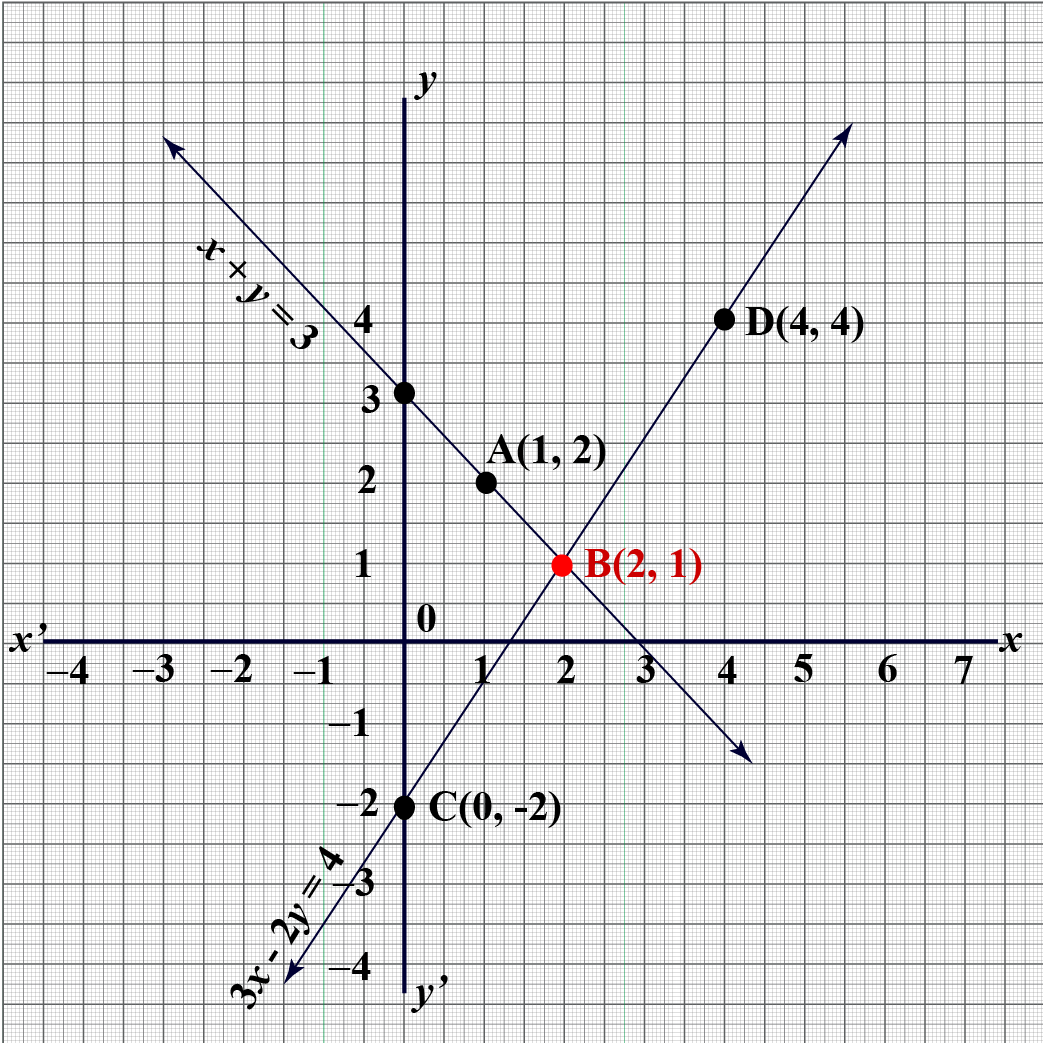# System of Equations Solver

System of Equations Solver

## It's riddle time!

James and John both go to a stationary shop. James buys 2 books and 1 file which costs him $35; John bought 3 books and 4 files of same cost for$65. If I want to buy 5 books and 2 files, what amount do I need to pay?

To find the answer to this, we need to use a system of linear equations.

In this mini-lesson we will learn about simultaneous equation solver, solving system of equations by elimination calculator, solving system of equations by substitution calculator, and simultaneous equations calculator in the concept of System of equations solver. Check out the interactive simulations to know more about the lesson and try your hand at solving a few interesting practice questions at the end of the lesson.Let's proceed to study the same!

## Lesson Plan

 1 What are System of Equations? 2 Tips and Tricks on Solving Linear Equations 3 Solved Examples on System of Equations 4 Challenging Questions on System of Equations 5 Interactive Questions on System of Equations

## What are System of Equations?

In the riddle that we mentioned in the introduction, we have two linear equations having two variables, cost of a book and the cost of a file.

Such equations are called system of equations.

"A system of linear equations is a set of equations which are satisfied by the same set of variables."

They are also known as simultaneous linear equations.

Let us understand how to solve these equations in detail

## Number Line

We can represent all real numbers on a number line.

### Horizontal Number Line

Numbers which are less that 0 lie on the left side of it and the one which are greater than 0 lie on its right side.### Vertical Number Line

While representing numbers on vertical line, the convension used is as follows:

Numbers which are less that 0 are placed below 0 and the one which are greater than 0 are placed above it.A linear equation in one variable can be plotted on a number line.

For example, we are given $$2x+ 6 = 0$$

To show this on a number line, we need to find its solution.

So, by transposition we get the solution which is$$\,x = -3$$This represents the point -3 on a number line.

## 4 Steps to Solving an Equation

To solve an equation, following steps are to be followed:

1. Simplify each side of equation.

Let us consider $$2x-9+3x= 3(x-2)$$

L.H.S$$=2x-9+3x=5x-9$$  and R.H.S$$= 3(x-2) =3x-6$$

2. Move like terms on either side.

So, the equation $$5x-9 =3x-6$$ on rearranging becomes

\begin{align} 5x-3x&=-6+9\\2x&=3 \end{align}

3. Make the coefficient of variable as 1

So, on dividing both sides by 2 we get

$$x=\dfrac{3}{2}$$

4. Check if the value of variable is satisfying the equation.

Plug in  $$x=\dfrac{3}{2}$$ in the equation $$2x-9+3x= 3(x-2)$$

\begin{align} \text{L.H.S} &=2x-9+3x\\&=2\times\dfrac{3}{2}-9 +3\times\dfrac{3}{2}\\&=5\times\dfrac{3}{2}-9\\&=\dfrac{15-18}{2}\\&=\dfrac{-3}{2}\\ \text{R.H.S} &=3(x-2)\\&=3(\dfrac{3}{2}-2)\\&=3 (\dfrac{3-4}{2})\\&=\dfrac{-3}{2}\end{align}

## 3 ways to Solve System of Equations

Let's understand 3 ways to solve an equation using examples.

### I. Substitution Method

Given two linear equations in x and y, express y in terms x in one of the equations and then substitute it in 2nd equation.

Consider

\begin{align} 3x-y=23\,...\,\,\,\,Eqn\,1\\ 4x+3y=48\,...\,\,\,\,Eqn\,2\end{align}

From(1), we get:

$$y=3x-23\,...\,\,\,\,Eqn\,3$$

Plug in y in (2),

\begin{align} 4x+3(3x-23)&=48\\ 13x-69&=48\\13x&=117\\\Rightarrow x&=9.\end{align}

Now, plug in $$x=9$$ in (1)

$$y=3\times9-23=4$$

Hence, $$x=9$$ and $$y=4$$ is the solution of given system of equations.

### II. Elimination Method

Here we eliminate one of the unknowns, by multiplying equations by suitable numbers, so as the coefficients of one of the variables become same.

Consider

$$2x+3y=4...\,\,Eqn(1)$$ and $$\,3x+2y=11...\,\,Eqn(2)$$.

The coefficients of y are 3 and 2; LCM (3,2) = 6

Multiplying Eqn(1) by 2 and Eqn(2) by 3, we get

\begin{align} 4x+6y&=8\,...\,Eqn(3)\\ 9x+6y&=33\,...\,\,Eqn(4)\end{align}

On subtracting (3) from (4), we get

\begin{align} 5x&=25\\\Rightarrow x&=5.\end{align}

Plugging in x = 5 in (2) we get

\begin{align} 15 +2y&=11\\\Rightarrow y&=-2.\end{align}

Hence, $$x=5,$$ $$y=-2$$ is the solution.

### III. Graphical Method

In this method, the solution of simultaneous equations is obtained by plotting their graphs.

"The point of intersection of the two lines is the solution of the system of equations."

Example: $$x+y=3\,$$and $$3x-2y=4$$

Find at least two values of x and y satisfying equation $$x+y=3$$

 x 1 2 y 2 1

So we have 2 points A (1,2 ) and B (2,1).

Similarly, find the at least two values of x and y satisfying equation $$3x-2y=4$$

 x 0 4 y -2 4

We have two points C(0,-2) and D( 4,4 ).
Plotting these points on the graph we get:We observe that the two lines intersect at (2,1). So, $$x = 2, y = 1$$ is the solution of given system of equations.

Methods I and II are the algebraic way of solving simultaneous equations and III is the graphical method.Tips and Tricks
1. Substitution method works best when any of the variable in one of the equations have coefficient 1
2. Elimination is easy to handle when we have system of equations with coefficients other than 1 and even more than 2 equations in the system.

## Solve System of Equations by using Algebra Calculator

Try solving some of the simultaneous equations and verify your answer using the simultaneous equations calculator.

### Solving System of Equations Solver

Use the methods given above and check your answer using the simultaneous equation solver provided below.

1. Solving System of Equations by Substitution Calculator

Input the coefficients for the two equations and see the answers using the calculator using substitution method.

2. Solving System of Equations by Elimination Calculator

Input the coefficients for the two equations and see the answers using the calculator using elimination method.

## Solving System of Equations using Graph

The solution of a system of linear equations in two variables can be obtained by plotting them on the Cartesian system.

Input the equations. Observe the graph obtained and find out the solution by locating the point of intersection.

## Solved Examples

 Example 1

In a $$\Delta LMN, \angle N = 3\angle M= 2(\angle L +\angle M).$$

With this information, James wants to know the angles of $$\Delta LMN.\,$$ Help him to find the answer.

Solution

Let $$\angle L = x^{\circ} \,and\, \angle M =y^{\circ}.$$

Then,

$$\angle N = 3\angle M =(3y)^{\circ}.$$

Now,

\begin{align} \angle L+\angle M+\angle N&=180 ^{\circ}\\\therefore x+y+3y&=180\\x+4y&=180\,...\,\,(1)\end{align}

Also,

\begin{align} \,\,\angle N&=2(\angle L+\angle M) \\\therefore 3y&=2(x+y)\\\Rightarrow2x-y&=0\,...\,\,(2)\end{align}

On multiplying (2) by 4 and adding the result to (1), we get

\begin{align} 9x&=180\\x&=20. \end{align}

Putting $$x=20$$ in (2), we get $$y=40$$ and $$3y = 120$$

 $$\therefore \angle L= 20 ^{\circ} , \angle M = 40^{\circ} \,and \,\angle N=120^{\circ}$$
 Example 2

Peter is three times as old as his son. 5 years later, he shall be two and half times as old as his son. What's Peter's present age?

Solution

Let Peter's age be $$x\,$$ years and his son's age be $$y\,$$years.

$$x=3y\,...\,Eqn(1)$$

5 years later,

Peter's age $$=x+5\,$$ years and his son's age $$=y+5\,$$years.

\begin{align} x+5&=\dfrac{5}{2}(y+5)\\2x-5y-15&=0 \,...\,Eqn(2)\end{align}

Put $$x=3y$$ in Eqn(2), we get

\begin{align}6y&=5y-15\\\therefore y&=15 \end{align}

Plug in $$y=15$$ in Eqn(1), we get

$$x=45$$

 $$\therefore$$ Present age of Peter = 45 years and Present age of son= 15 years
 Example 3

Tressa starts her job with a certain monthly salary and earns a fixed increment every year. If her salary was $1500 after 4 years and$1800 after 10 years of service, find her starting salary and annual increment.

Solution

Let her starting salary be $$x$$ and annual increment be $$y$$.

After 4 years, her salary was $1500 $$x+4y=1500\,...\,Eqn\,(1)$$ After 10 years, her salary was$1800

$$x+10y=1800\,...\,Eqn\,(2)$$

Subtracting Eqn (1) from (2), we get

\begin{align}  6y&=300\\\therefore y&=50\end{align}

On putting $$y=50$$ in Eqn (1), we get $$x=1300$$

 $$\therefore$$Starting salary was $1300 and annual increment is$50
 Example 4

Jason is asked to find the solution to the following simultaneous equations graphically.

$$2x-y-4=0$$

$$x+y+1=0$$

Help Jason to reach the solution.

Solution

To find the graph of the given equations, we will write y in terms of x and find at least two solutions of each of the equations.

\begin{align}   2x-y-4&=0\\\therefore y &= 2x-4\end{align}

 x 0 2 y -4 0

\begin{align}   x+y+1&=0\\\therefore y &= -x-1\end{align}

 x 0 -1 y -1 0

Now, we proceed to plot these points on a graph.The point of intersection gives the solution of the simultaneous equations.

 $$\therefore$$ Solution is $$x=1,\,y=-2$$Challenging Questions
1. What will be the length of each side of an equilateral triangle with sides $$(x +3y)$$inches, $$(4x + \dfrac{y}{2} +1)$$inches and $$(3x+2y-2)$$inches.
2. Which method would you prefer to solve the system of equations and why?

$$152m-378n=-74$$ and $$-378m+152n=-64$$

## Interactive Questions

Here are a few activities for you to practice.

Select/Type your answer and click the "Check Answer" button to see the result.

## Let's Summerize!

We hope you enjoyed learning about System of Equations Solver with the simulations and practice questions. Now, you will be able to easily solve problems on 4 simultaneous equation solver, solving system of equations by elimination calculator, solving system of equations by substitution calculator, and simultaneous equations calculator. The math journey around system of equations starts with what a student already knows, and goes on to creatively crafting a fresh concept in the young minds. Done in a way that not only it is relatable and easy to grasp, but also will stay with them forever. Here lies the magic with Cuemath.

## About Cuemath

At Cuemath, our team of math experts is dedicated to making learning fun for our favorite readers, the students!

Through an interactive and engaging learning-teaching-learning approach, the teachers explore all angles of a topic.

Be it worksheets, online classes, doubt sessions, or any other form of relation, it’s the logical thinking and smart learning approach that we at Cuemath believe in.

## 1. How do you create system of equation with two variables?

To create a system of equations with two variables:

• First, identify the two unknown quantities in the given problem.
• Next find out the two conditions given and frame equations for each of them.

With this two steps, a system of equations with two variables gets created.

## 2. How do you solve 2 equations with 3 variables?

An equation with 3 variables represent a plane.

Step 1) To solve a system of 2 equations with 3 variables say x, y and z, we will consider the 1st two equations and eliminate one of the variable, say x, to obtain a new equation.

Step 2) Next, we write the 2nd variable, y in terms of z from the new equation and substuite it in the third equation.

Step 3) Assuming z = a, we will obtain values of x and y also in terms of a.

Step 4) Once, we know the value of a, we can find the values of x, y and z.

More Important Topics
Numbers
Algebra
Geometry
Measurement
Money
Data
Trigonometry
Calculus